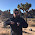Sunday, August 25, 2013

The interest rate in the information transfer model

This post is a first step towards integrating the information transfer versions of the IS-LM model and the quantity theory. We'll begin with one of the basic equations from the information transfer framework:

$$P = \frac{1}{\kappa}\frac{Q^d}{Q^s}$$

In the LM market, we have aggregate demand represented by $NGDP$ as an information source sending information to the money supply (the monetary base, $MB$) with the interest rate as the price (the detector that detects the signal transmitted from the demand to the supply). We'll write this price $P \rightarrow r$ as

$$c \log r = \log \frac{1}{\kappa}\frac{NGDP}{MB}$$

With $c$ being an arbitrary constant. If I fit this equation to the Effective Fed Funds rate, I get a very good fit (model is blue, data is green):

The fit parameters are $1/\kappa = 39.1$ and an overall normalization of $c = 0.279$ (assuming of course that the fed funds rate is divided by 100 to change from a percentage into a dimensionless number).

An interesting way to visualize this data is to plot the interest rate versus real GDP (aka real output, denoted $Y$ in the ISLM model). With the information transfer model providing both the interest rate (above) and RGDP derived from $NGDP$ and the price level in the quantity theory, we can observe "LM curves" in the data where increasing $Y$ traces out an upward sloping curve with the interest rate.

It appears as though the LM curves are "reset" by the Fed lowering interest rates to "heat" the economy (by increasing the monetary base via the equation above). There was a period of relative constant interest rates (dashed red line) from the mid-90s to the early 2000s (the late 90s "tech boom") where the economy grew with limited intervention from the Fed. That last statement would probably send Scott Sumner up the wall. The Fed is always intervening, but in this case by limited intervention I mean keeping the monetary base growing roughly at the same rate as NGDP. This keeps interest rates constant via the equation above.

In 2008 we see the data bump up against the zero lower bound. The LM market stops sending information detectable by the interest rate. Here we would obtain the "flat" LM curve of the liquidity trap. This is different from the constant interest rate of the late 1990s, which is the LM market equilibrium moving along at roughly a constant interest rate as $Y$ increases until 2001.

It appears there is a qualitative change in the properties of the LM market that begin in the early 1990s -- interestingly the first recession with Alan Greenspan as Fed chair. For some reason, no LM curve appears after the recession ends. Could raising interest rates in the late 1990s have helped us avoid the Great Recession later on by leaving interest rates high enough to avoid the zero lower bound?

Should proper macroeconomic stabilization produce a picture that looks (heuristically) like this:

And do the 90s look like a massive failure in retrospect?

The next step (next post maybe?) is to see if using the real interest rate (related via the Fisher equation to the nominal rate) shows any significant difference in qualitative behavior.

1.By "cool" I meant "heat"; originally wrote the sentence referring to the rising LM curves ...

2.I mention "integrating ... the IS-LM model and the quantity theory" at the top of the post. Brad DeLong has a great description of how these models relate:

One way to think about the second to last graph is that it plots out the changing LM curves DeLong describes.

3.Sorry, I'm not quite sure how you end up with the equation for r. Based on r:NGDP->MB, isn't the equation for r = dNGDP/dMB = 1/k (NGDP/MB) - which should net you the exact same form as P:NGDP->MB (P = 1/k (MB/Mo)^(1/k-1))?

1.Great question! I don't think I ever really explained that anywhere on the blog. The price level model P:NGDP→MB takes both information source (NGDP) and destination (MB) to be "endogenous" while the interest rate model r:NGDP→MB takes the information source and destination to be "exogenous" (they come from outside the model).

http://informationtransfereconomics.blogspot.com/2013/10/exogenous-and-endogenous.html

Additionally, the equation Price = (1/k) (Demand/Supply) should hold generally (the "endogenous" solution just takes "Demand" to be "Supply^1/k").

There is another piece: the constant c above doesn't come from anywhere and is something I'm still trying to figure out:

http://informationtransfereconomics.blogspot.com/2014/03/unsolved-problems-in-information.html